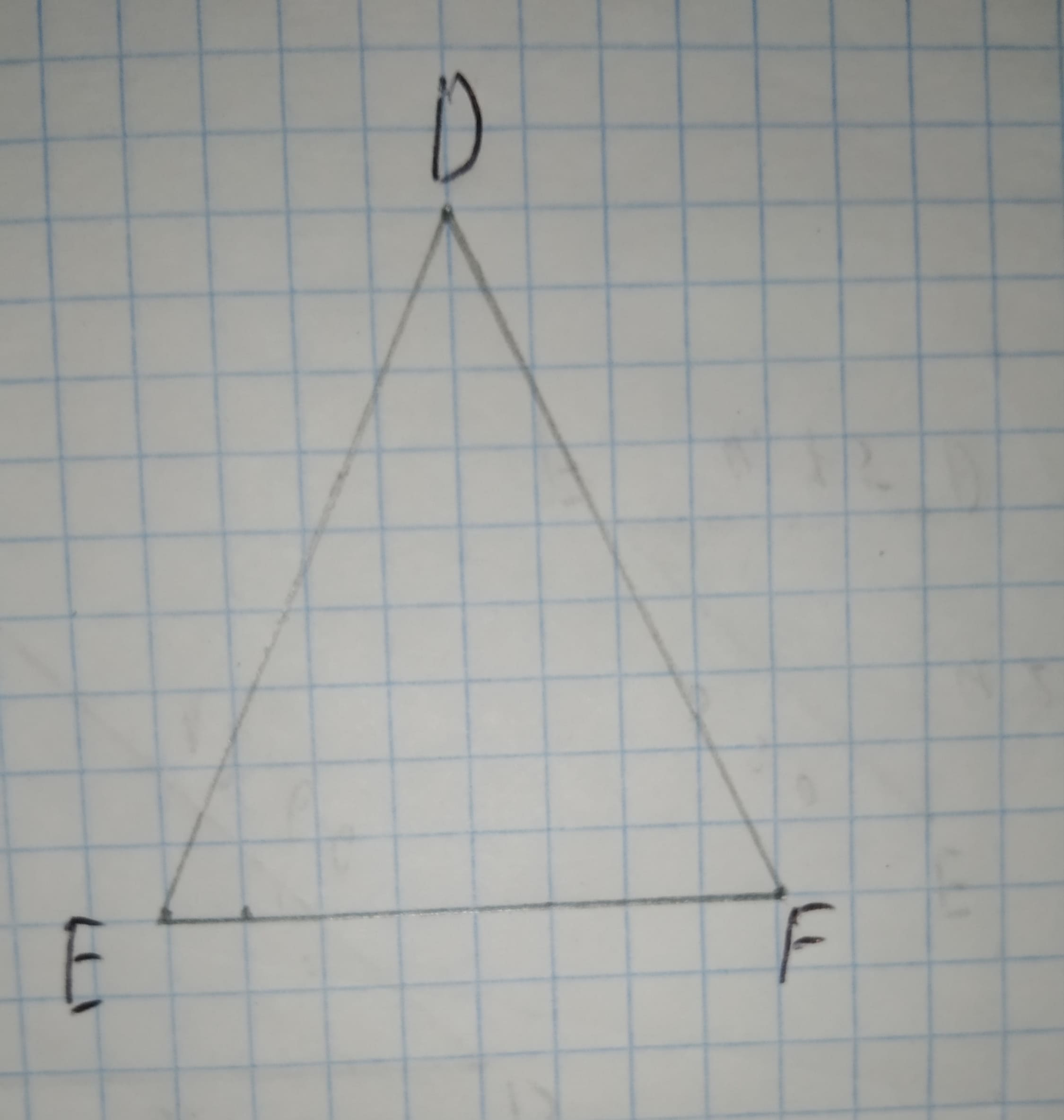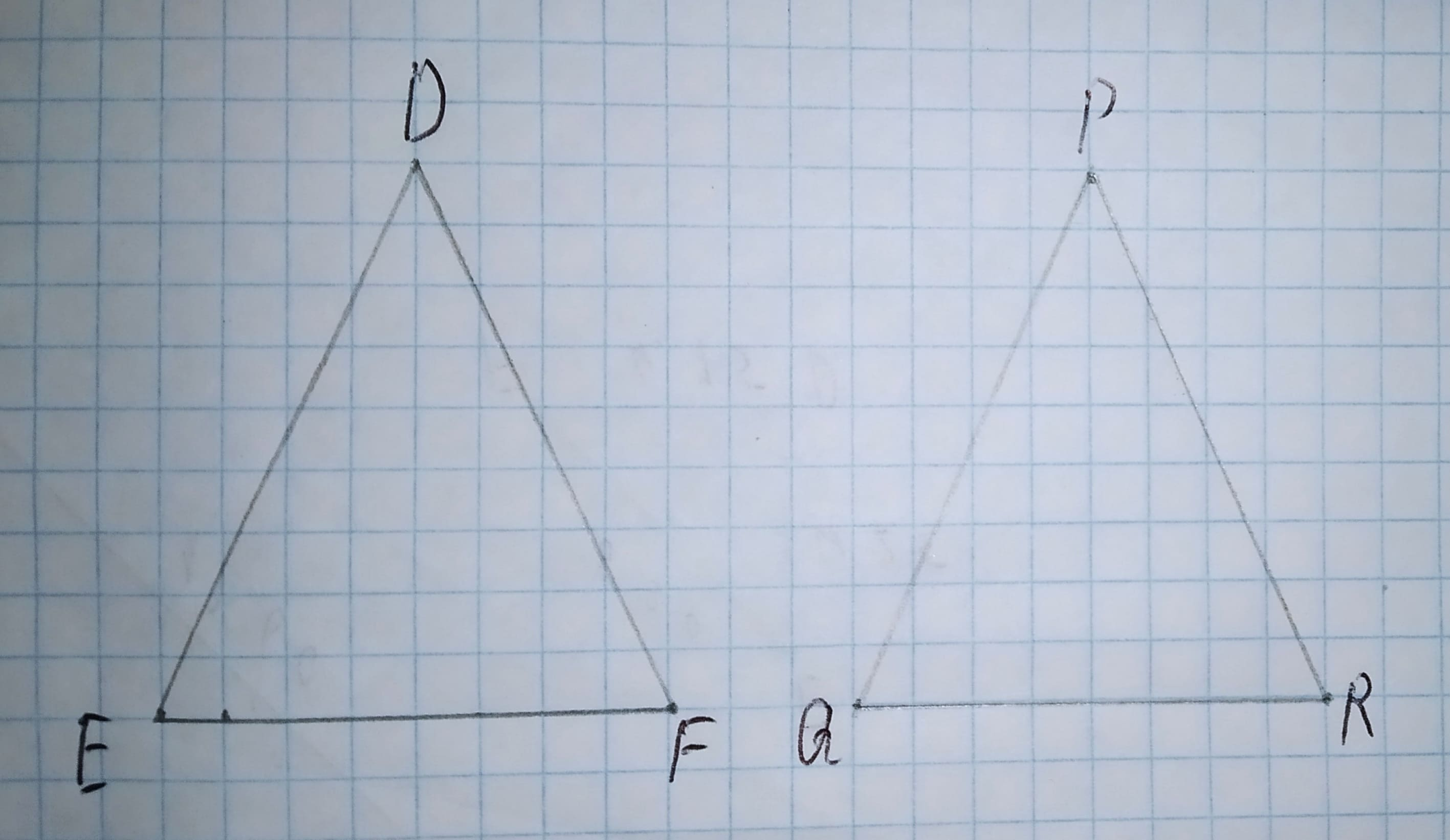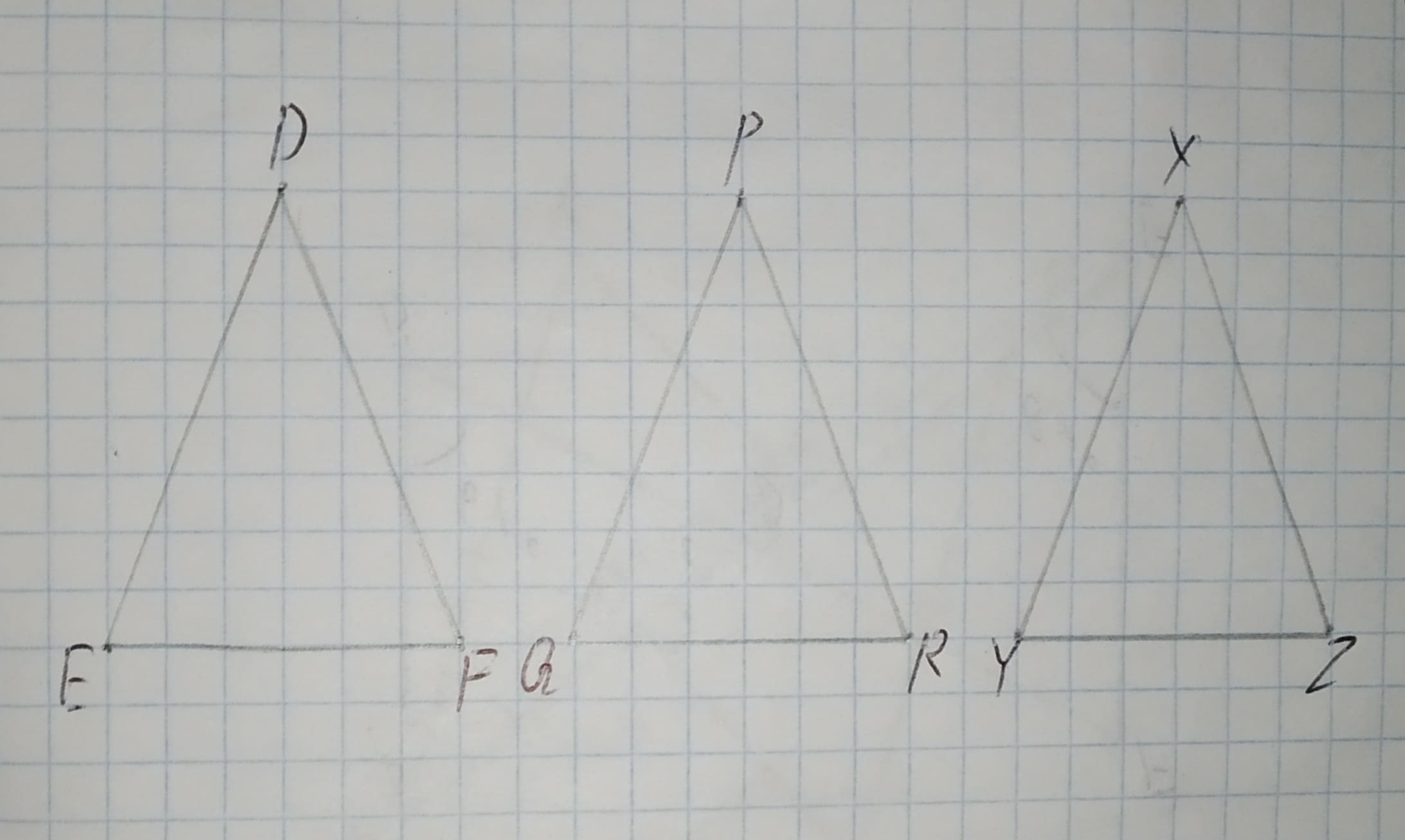# Does congruence of triangles have the reflexive property? the symmetric property? the transitive property?hexacordoK 2021-01-10 Answered
Does congruence of triangles have the reflexive property? the symmetric property? the transitive property?
You can still ask an expert for help

## Want to know more about Congruence?

• Questions are typically answered in as fast as 30 minutes

Solve your problem for the price of one coffee

• Math expert for every subject
• Pay only if we can solve itjlo2niT

Step1
Given:
The congruence of triangles.
Step 2
To identify whether the congruence of triangles have the reflexive property, the symmetric property, and the transitive property.
i) The reflexive property of a triangle:
Consider, $\mathrm{△}DEF$$⇒\mathrm{△}DEF\stackrel{\sim }{=}\mathrm{△}DEF$.
Hence, the congruence of triangle have the reflexive property.
Step 3
ii) The symmetric property of a triangle:
Consider, $\mathrm{△}DEF\phantom{\rule{1em}{0ex}}\text{and}\phantom{\rule{1em}{0ex}}\mathrm{△}PQR$For, $\mathrm{△}DEF\stackrel{\sim }{=}\mathrm{△}PQRthen\mathrm{△}PQR\stackrel{\sim }{=}\mathrm{△}DEF$.
Hence, the congruence of triangle have the symmetric property.
Step 4
iii) The transitive property of a triangle:
Consider, $\mathrm{△}DEF\phantom{\rule{1em}{0ex}}\text{and}\phantom{\rule{1em}{0ex}}\mathrm{△}PQR$For, $\mathrm{△}DEF\stackrel{\sim }{=}\mathrm{△}PQR\phantom{\rule{1em}{0ex}}\text{and}\phantom{\rule{1em}{0ex}}\mathrm{△}PQR\stackrel{\sim }{=}\mathrm{△}XYZthen\mathrm{△}DEF\stackrel{\sim }{=}\mathrm{△}XYZ$.
Hence, the congruence of triangle have the transitive property.